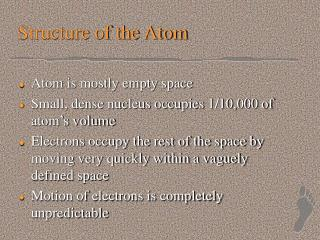DownloadDownload PresentationStructure of the Atom

# Structure of the Atom

Download Presentation## Structure of the Atom

- - - - - - - - - - - - - - - - - - - - - - - - - - - E N D - - - - - - - - - - - - - - - - - - - - - - - - - - -
##### Presentation Transcript

1. Structure of the Atom • Atom is mostly empty space • Small, dense nucleus occupies 1/10,000 of atom’s volume • Electrons occupy the rest of the space by moving very quickly within a vaguely defined space • Motion of electrons is completely unpredictable

2. Subatomic particles • In the nucleus • Protons: +1 charge, 1 amu mass (1.67x10-24g) • Neutrons: 0 charge, 1 amu mass • Protons normally repel - at close range the nuclear strong force takes over • Around the nucleus, in energy levels • Electrons: -1 charge, 1/1840 amu (9.1x10-28g)

3. Atomic Identity • Atoms of an element are identified by atomic number = number of protons in the nucleus 6 atomic number (whole number) C 12.011 carbon

4. Atomic identity • Mass number = #protons + #neutrons • Applies only to individual atoms • # neutrons can vary among atoms of an element • Atoms of same element with different # neutrons: ISOTOPES

5. Isotopes and mass number • Isotopes are identified by name and mass number carbon-13 13C Carbon-13 has 7 neutrons (13-6)

6. Example problems • What is the name of an isotope that has 17 protons and 18 neutrons? • Chlorine-35 • How many neutrons are in an atom of uranium-235 (U-235)? • 235-92 = 143

7. Example problems • One atom has 14 protons and 15 neutrons, and another has 13 protons and 15 neutrons. Are they isotopes? Why or why not? • They are not isotopes. Because they have different numbers of protons, they are different elements.

8. Review • Atomic number = number of protons • Mass number = number of protons + number of neutrons • Isotopes are atoms of the same element with different mass numbers • Same number of protons • Different number of neutrons

9. Average atomic mass • Masses of individual atoms 1 amu = 1/12 mass of 12C 1 proton = 1.007276 amu 1 neutron = 1.008665 amu 1 electron = 0.000549 amu • Except for 12C, masses of elements are usually not whole numbers

10. Average atomic mass • Relative abundance of isotope = amount of isotope ÷ total amount of element • Relative abundances are fairly constant in naturally occurring elements • Average atomic mass is the weighted average of all the masses of all the atoms of an element

11. Average atomic mass • Average atomic mass is displayed on the periodic table 6 average atomic mass (not a whole number) C 12.011 carbon

12. Calculating average atomic mass • Each isotope’s mass must be multiplied by its relative abundance. • If the abundance is written as a decimal, then the total items is “1”. • The average atomic mass is the sum of the abundance x mass for each isotope

13. Calculating average atomic mass • Example: Chromium Isotope mass abundance % 50Cr 49.9460464 4.345 52Cr 51.9405098 83.789 53Cr 52.9406513 9.501 54Cr 53.9388825 2.365

14. Calculating average atomic mass 50Cr 49.9460464 x 0.04345 = 2.170 52Cr 51.9405098 x 0.83789 = 43.520 53Cr 52.9406513 x 0.09501 = 5.030 54Cr 53.9388825 x 0.02365 = 1.276 + Sum: 51.996 amu